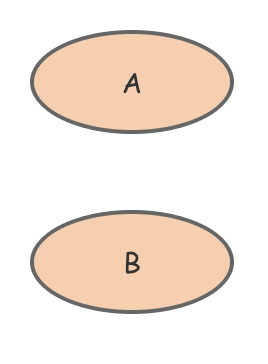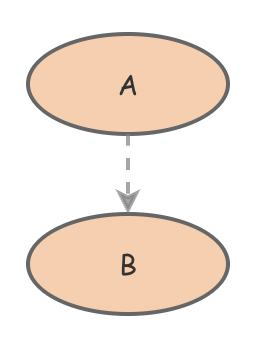A link makes two primitives (flows, stocks, converters, or parameters) aware of each other. This allows the two primitives to reference the value of each other in their equations. A flow is automatically aware of both its Alpha (start) and Omega (end). In other cases, if two primitives are not connected by a link, you will be unable to reference the value of the first in the equations of the second or vice versa.

For an example of the use of links, take the following model that only contains two parameters: A and B. A has some arbitrary value that is not important to us. B’s value is set to the following (the carrot sign “^” means to carry out a power operation, so the function here returns the square of the value of A):

[A]^2

We could construct our model like this:When we run this model though, Insight Maker will print out an error that no connection to A was found from primitive B. This is because A and B are not connected in any way so B is unaware that A exists. Therefore, you cannot reference the value of A from B. This problem is easy to fix though; just create a link joining A and B like this:This time, the model runs perfectly.